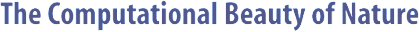Computer Explorations of Fractals, Chaos,Complex Systems, and Adaptation· title page · home* · cover artwork · jacket text · table of contents · the author* · ordering informationBook Contents
 · three themes · part synopses · selected excerpts · all figures from book · quotes from book · glossary from book · bibliography · slide showSource Code
 · overview &documentation · FAQ list* · download source code · java appletsMiscellany
 · news* · reviews & awards · errata · for educators · bibliography (BibTeX format) · other linksPREDPREY Documentation

```

```

#### NAME

```       predprey - plot the phase space of a predator-prey system

```

#### SYNOPSIS

```       predprey -help
or
predprey
[-width  integer] [-height integer] [-skip integer]
[-points integer] [-alpha double] [-delta  integer]
[-dt double] [-x0 double] [-y0 double] [-z0 double]
[-data] [-xp string] [-yp string] [-factor  double]
[-inv] [-mag integer] [-term string]

```

#### DESCRIPTION

```       The  phase  space of a three species predator-prey system,
which is described by the three differential equations

dx/dt = x * (1.1 - x / 2 - y / 2 - z / 10),

dy/dt = y * (-0.5 + x / 2 + y / 10 - z / 10), and

dz/dt = z * (alpha + 0.2 - alpha * x - y / 10 - z / 10),

is plotted according to the specified  parameters.   Valid
arguments  passed  with the -xp and -yp options can be any
one of x(t), y(t), z(t), x(t-delta), y(t-delta),  or  z(t-
delta).   Thus,  the displayed plot can take the form of a
state space plot or a delayed coordinate plot.

```

#### OPTIONS

```       -width integer
Width of the plot in pixels.

-height integer
Height of the plot in pixels.

-skip integer
Number of initial points to skip.

-points integer
Number of points to plot.

-alpha double
Value of the alpha parameter.

-delta integer
Time delay term.

-dt double
Time step.

-x0 double
Initial X value.

-y0 double
Initial Y value.

-z0 double
Initial Z value.

-data  Don't plot, but print points.

-xp string
X-coordinate for plot.

-yp string
Y-coordinate for plot.

-factor double
Auto-scale expansion factor.

-inv   Invert all colors?

-mag integer
Magnification factor.

-term string
How to plot points.

```

#### MISCELLANY

```       The plot region is determined by the points that are  ini-
tially  skipped.  If this number is too small (i.e., it is
not very representative of the range of the  plotted  val-
ues),  then  you may need to increase the number specified
by the -skip option.  Alternatively, you  can  adjust  the
value   given   to   -factor,  which  simply  fractionally
increases the border of the plot.

The program uses a second-order Euler's method to  perform
the  numerical integration, which is sufficient for simple

```

#### BUGS

```       No sanity checks are performed to make sure  that  any  of
the options make sense.

```

#### AUTHOR

```       Copyright (c) 1997, Gary William Flake.

Permission  granted  for any use according to the standard
GNU ``copyleft'' agreement provided that the author's com-
ments  are  neither  modified nor removed.  No warranty is
given or implied.

```Copyright © Gary William Flake, 1998-2002. All Rights Reserved.Last modified: 30 Nov 2002